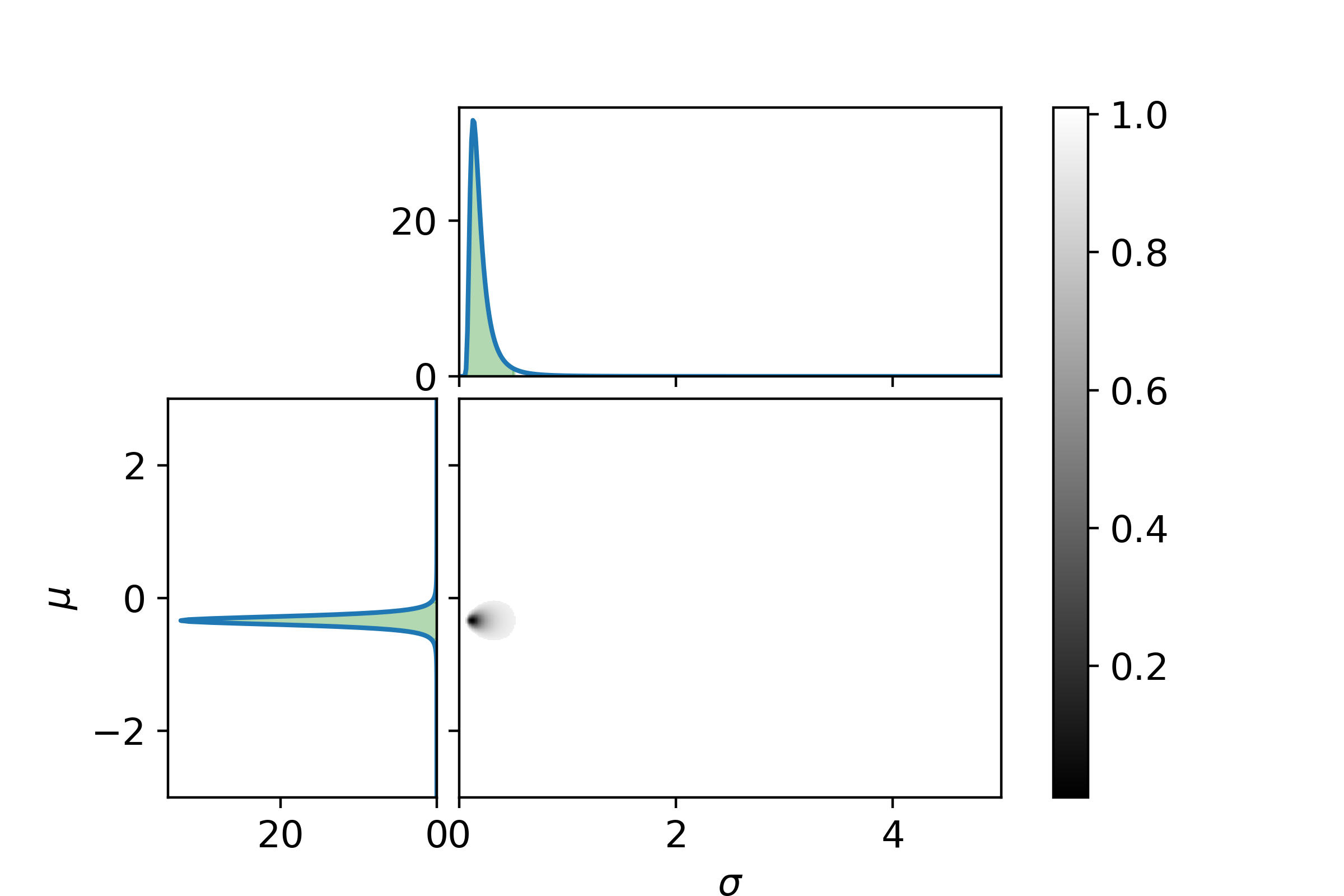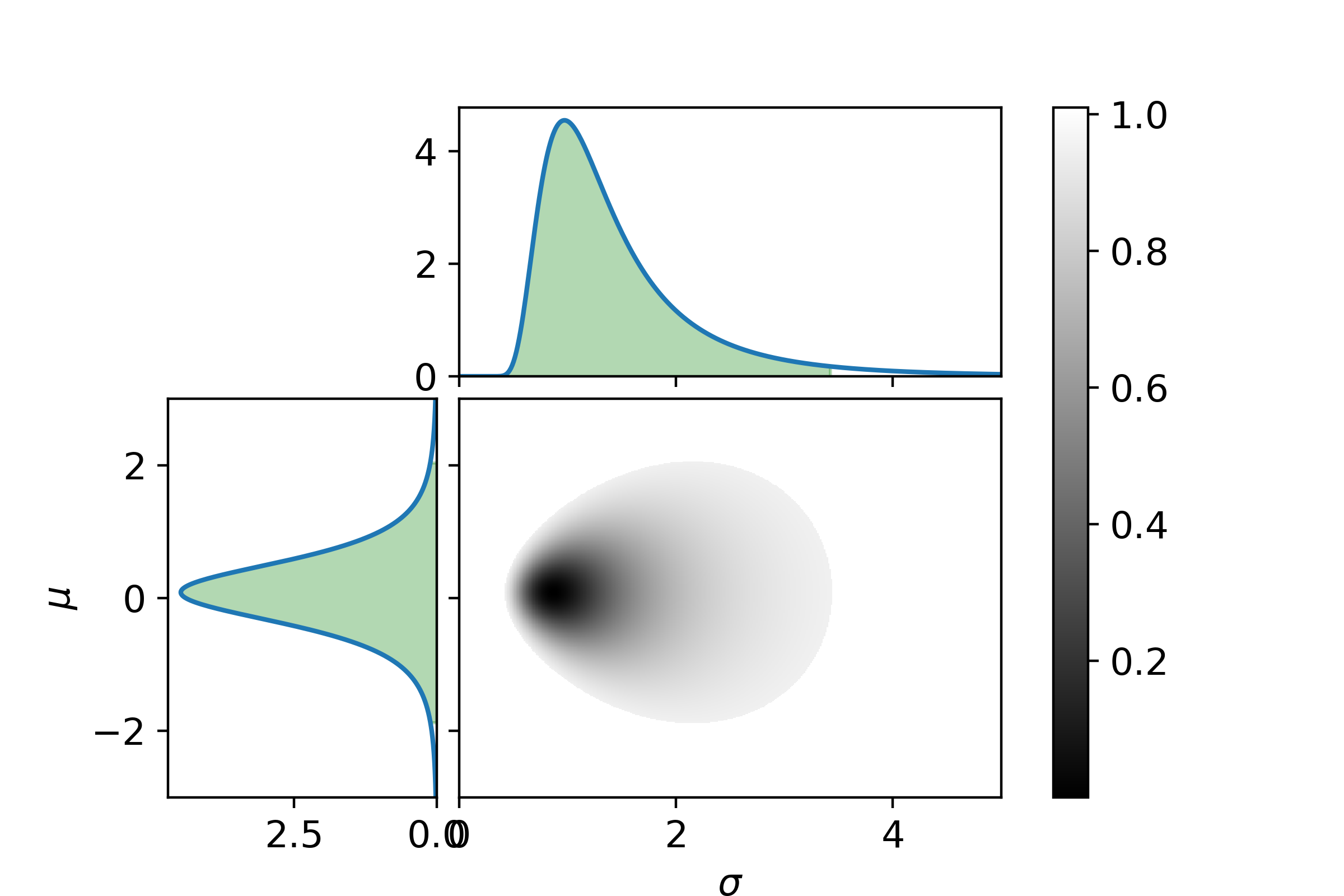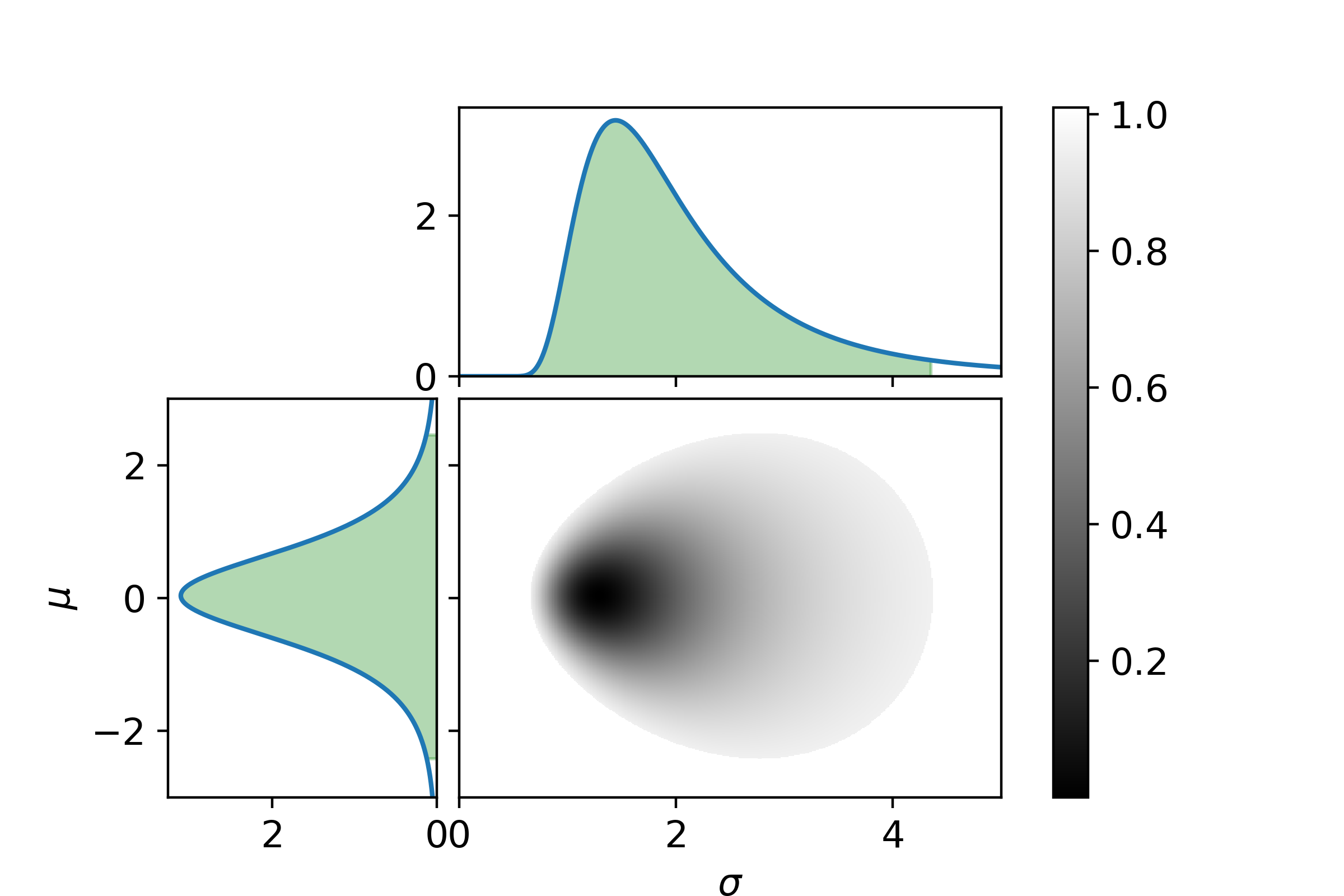# Benchmark tracking certainty of funds

In the decision of which funds to invest in, an important parameter is how well the fund can track the benchmark index. It is to be expected that variance of how well a specific fund can track an index does not go to zero even if we had infinite data. As a simpel model the tracking of the benchmark could be modeled as a Gaussian distribution. This gives two free parameters. The mean value, $$\mu$$, how close the fund tracks the benchmark on average. And the standard deviation, $$\sigma$$, how much the fund deviates from the benchmark at specific points in time.

The mean and standard devitation has been calculated for specific funds, see this Reddit post. These quantities are very easy to determine (without error bars). Now let us take this one step further, and give an estimate of the certainty of the mean and standard devitation, to find out if we can actually distinguish the perfermance of these specific funds.

The data from the Reddit post is the following:

Year DKIGI deviations SPVIGAKL deviations SPIEMIKL deviations
2013 -0.28 - -
2014 -0.28 - -0.94
2015 -0.52 - -1.34
2016 -0.42 - 2.37
2017 -0.19 - -0.07
2018 -0.20 -0.54 0.16
2019 -0.40 1.06 0.96
2020 0.34 1.01 -2.02
2021 -0.11 -1.17 0.27
2022 -0.82 -0.07 2.63

In a previous post it was shown how to use Bayesian inference to get a likelihood distribution of the parameters. Now using Bayesian inference on the data represented above gives the following graphs.

For DKIGIFor SPVIGAKLFor SPIEMIKLIt can clearly be seen that how well DKIGI follows the bechmark is much more determined than for the other two. Now to make it easier to compare, let us look at the 95% confindence and the maximum likelyhood for the mean values and standard deviations.

 DKIGI $$\mu_\mathrm{max\ likelihood}\in\{\mu_\mathrm{max},\ \mu_\mathrm{min}\}$$ SPVIGAKL $$\mu_\mathrm{max\ likelihood}\in\{\mu_\mathrm{max},\ \mu_\mathrm{min}\}$$ SPIEMIKL $$\mu_\mathrm{max\ likelihood}\in\{\mu_\mathrm{max},\ \mu_\mathrm{min}\}$$ $$-0.34 \in \{-0.04, -0.62\}$$ $$0.08 \in \{2.05,-1.87\}$$ $$0.04 \in \{2.47, -2.41\}$$
 DKIGI $$\sigma_\mathrm{max\ likelihood}\in\{\sigma_\mathrm{max},\ \sigma_\mathrm{min}\}$$ SPVIGAKL $$\sigma_\mathrm{max\ likelihood}\in\{\sigma_\mathrm{max},\ \sigma_\mathrm{min}\}$$ SPIEMIKL $$\sigma_\mathrm{max\ likelihood}\in\{\sigma_\mathrm{max},\ \sigma_\mathrm{min}\}$$ $$0.11 \in \{0.51, 0.06\}$$ $$0.87 \in \{3.43,0.43\}$$ $$1.29 \in \{4.36, 0.67\}$$
If you enjoyed this post you can donate a coffee , if you like :)